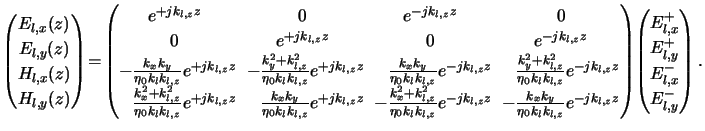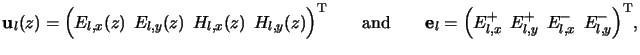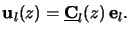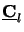Next: Matrix Factorization Up: C.1 One Homogeneous Planar Previous: Magnetic Field

### Matrix Notation

We introduce now a compact matrix notation for the two relations (C.5) and (C.7) by combining them like(C.6)

Summarizing the z-dependent part of the lateral components of the electric and magnetic field in ul(z) and, similarly, the electric amplitudes propagating upwards and downwards the layer in el, i.e.,(C.7)

we simply obtain(C.8)

The matrix(z) definitely describes the field propagation in the layer l, i.e., the electric and magnetic field can be calculated at any vertical position z within the layer from the above equation (C.10).

Heinrich Kirchauer, Institute for Microelectronics, TU Vienna
1998-04-17